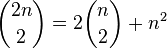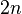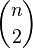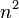# 15-344/Homework Assignment 8

This assignment is due at the tutorials on Thursday November 26. Here and everywhere, neatness counts!! You may be brilliant and you may mean just the right things, but if the teaching assistants will be having hard time deciphering your work they will give up and assume it is wrong.

9a) Show by combinatorial argument that$\binom{2n}{2} = 2 \binom{n}{2} + n^2$
A)Split$2n$ into two groups of n. Then choosing 2 out of$2n$ can be seen as either choosing 2 from group 1 only , which is$\binom{n}{2}$ or 2 from group 2 only, which is also$\binom{n}{2}$, or one from each group, which is$n^2$. Their sum gives the total number of possibilities, which is exactly the right hand side of the equality.